Labeling Voltages, Currents, and Nodes

As discussed in the previous section, Kirchhoff's Voltage Law and Kirchhoff's Current Law are rules that describe how to form current and voltage equations from the connected graph of a circuit. In essence, they are bookkeeping or accounting rules stating how charge and potential energy must balance.

The rules themselves aren't very complicated, but -- like bookkeeping -- they quickly become confusing to electronics beginners because of two issues:

1. Labeling voltages and currents consistently, and
2. Keeping track of the sign or direction consistently.

Individual Components

We can label every two-terminal component with a voltage and a current. The usual convention is to define current as positive when going into the positive terminal of a circuit element: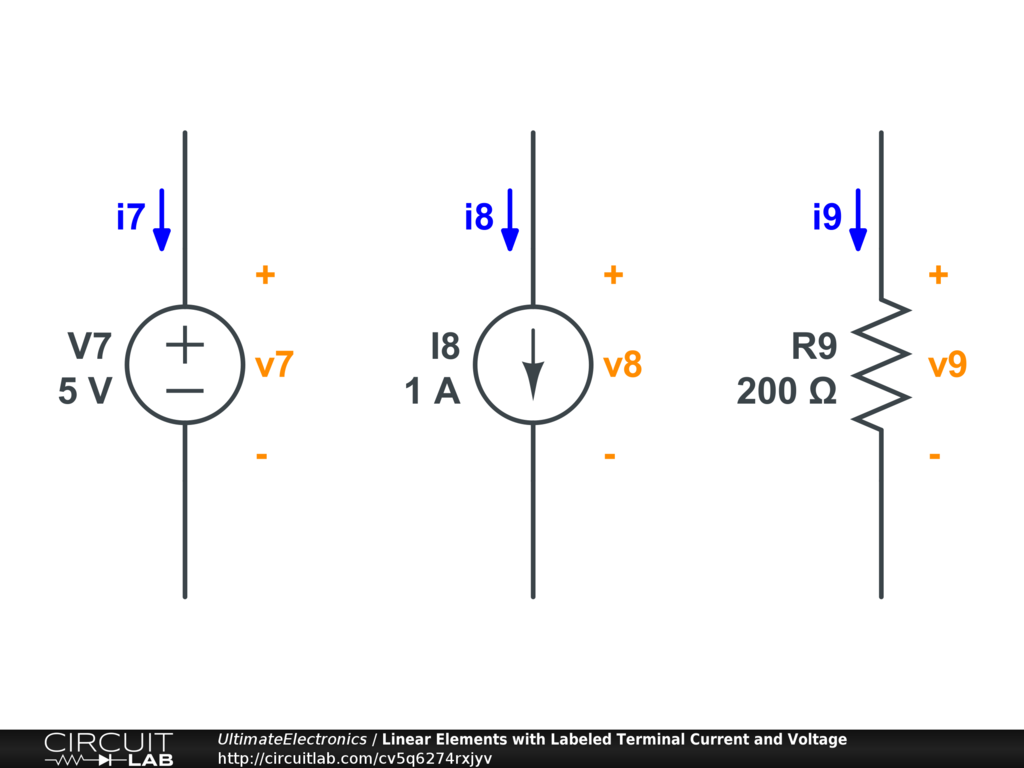For a voltage source, the positive terminal is clearly defined by the $+$ sign on the schematic symbol. The current is defined as positive going into the $+$ terminal.

For a current source, the symbol shows a pointy head and a thin tail to the arrow indicating the direction of current flow. In the drawing shown here, we choose to define the positive terminal as the tail of a current source. This is convenient because if we again define the current as positive going into that terminal, then the current is the same as the defined value of the source.

For a resistor, there is no directionality to the component. Nonetheless, we indicate a positive and negative terminal for the voltage $v_9$ as shown, and we indicate a direction for the current $i_9$. Our choice of which terminal to call $+$ was arbitrary, but as long as the current $i_9$ is defined as positive going into that terminal, then Ohm's Law $v_9 = i_9 R_9$ will apply without a change in sign. (If we flip the direction of the current $i_9$ without re-labeling the $+$ terminal, we'd have to write $v_9 = - i_9 R_9$.)

The convention to label current as positive when flowing into the $+$ terminal is arbitrary, but it makes a few things work out simply and consistently:

1. First, V-I relationships like the definition of Ohm's Law don't need a minus sign.
2. Second, power consumption is defined correctly with $P = v i$, where $P > 0$ for ordinary components which are consuming power like a resistor, and $P < 0$ for components which are supplying power like a battery.

Terminal Voltages and Currents

When solving a circuit on paper, we can choose to label currents and voltages around the schematic with whatever names we like, as long as we take care to keep track of their direction and sign.

But when solving using circuit simulation software like CircuitLab, each terminal of every component has a built-in name that can be used to reference its current. In this simple example, we've labeled these currents explicitly: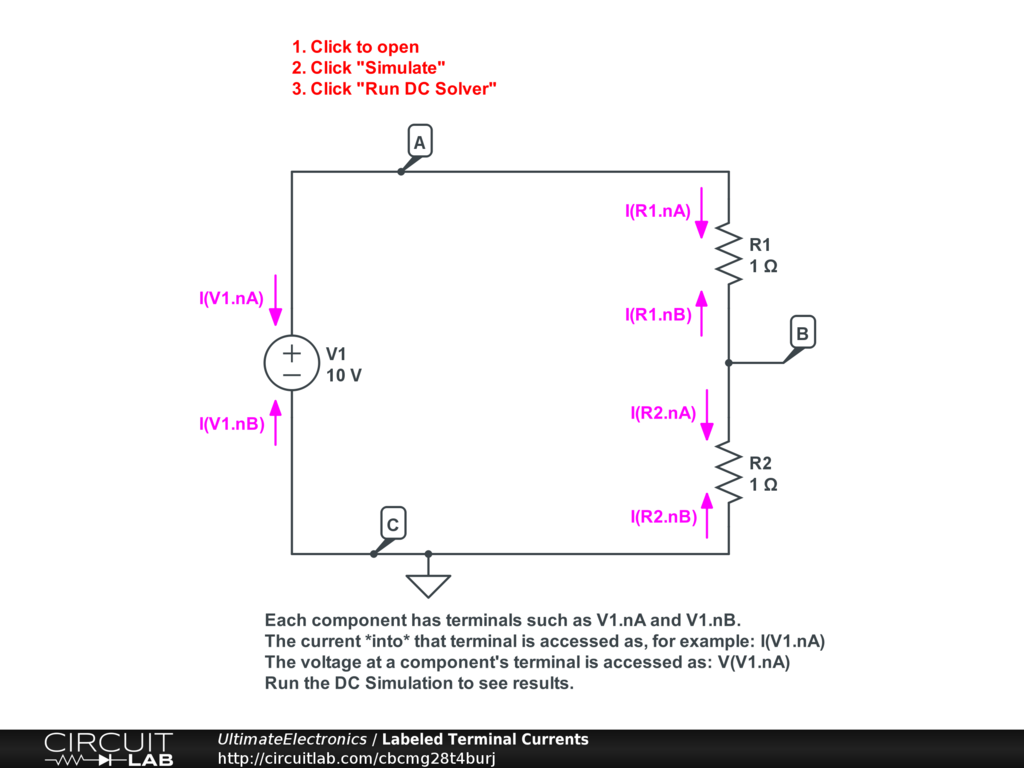A component such as voltage source "V1" has two terminals named "V1.nA" and "V1.nB". We can ask the simulator to show the current into a particular terminal by asking for "I(V1.nA)" for example. We can also show the voltage at a terminal with respect to ground by asking for "V(V1.nA)" for example.

For components like resistors it may not be obvious which terminal is "nA" and which is "nB". However, if you run a DC simulation and then mouse around the schematic, you'll be able to hover your mouse cursor over each individual terminal, and it will show the terminal name, voltage, and current.

You can also add custom expressions to calculate, such as "V(R1.nA)-V(R1.nB)", which will calculate the voltage drop across resistor R1.

You are encouraged to click the circuit above, run this simple simulation, and play around with various expressions to identify currents and voltages in the circuit.

Within the example circuit shown above, a number of equalities are present because we have several names for the same quantities.

For example, for all two-terminal components, the current into one terminal must come out of the other terminal, so:

\begin{align} \text{I(V1.nA)} & = - \text{I(V1.nB)} \\ \text{I(R1.nA)} & = - \text{I(R1.nB)} \\ \text{I(R2.nA)} & = - \text{I(R2.nB)} \end{align}

(Note that numerically it happens that there is only a single current value in this circuit because it is a simple single loop, but this will not always be the case.)

The voltages at each node in the circuit can be referenced either as one or more component terminals that connect to that node, or as an explicitly named node (see labels "A", "B", and "C") above. Referencing them in any of these ways is identical to the simulator, but for your convenience you are encouraged to label useful nodes where appropriate. And for ground, those voltages are automatically set equal to zero:

\begin{align} \text{V(A)} & = \text{V(V1.nA)} = \text{V(R1.nA)} \\ \text{V(B)} & = \text{V(R1.nB)} = \text{V(R2.nA)} \\ \text{V(C)} & = \text{V(V1.nB)} = \text{V(R2.nB)} = 0 \end{align}

In the previous section on Kirchhoff's Voltage Law we discussed identifying different paths and how the notation $v_{A B}$ indicates the voltage at node A with respect to node B. In the simulation environment, all individual named nodes are referenced with respect to ground. So, if we want to solve for a node-relative voltage in CircuitLab, we have to write an expression:

$$v_{A B} = \text{V(A) - V(B)}$$

Here's step-by-step instructions to solving for $v_{A B}$:

1. Click the schematic image for "Labeled Terminal Currents" a few paragraphs above. The CircuitLab software will open in a new browser tab.
2. At the bottom of the screen, click Simulate to switch into simulation mode.
3. In the DC tab, click the + Add Expression button. Type in "V(A) - V(B)" (without the quotes, but with the capitalization!) and press enter. This expression will be added to the list.
4. Click the Run DC Solver button.

Follow along to make sure you have proficiency in using the simulation environment to identify specific currents and voltages.

Multi-Terminal Components

Let's consider a slightly more complicated example: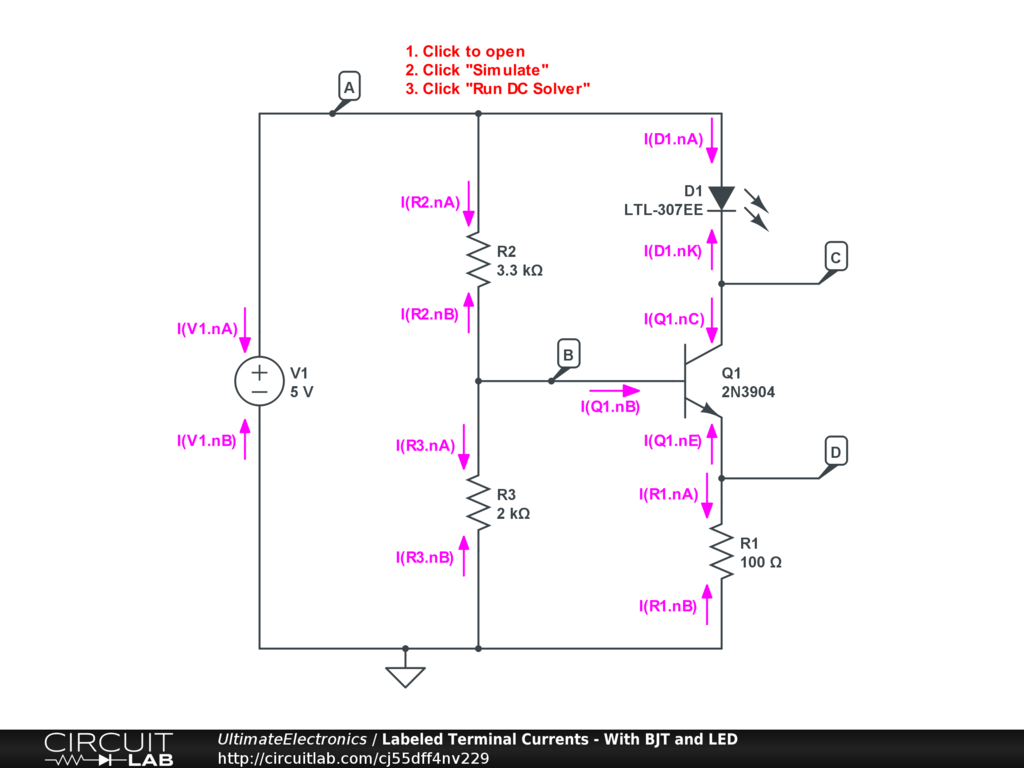Interactive Exercise Click to open and simulate the circuit above.

This circuit has 5 nodes (labeled A, B, C, D, and ground), and 6 elements.

The terminals of the LED named D1 are named "D1.nA" and "D1.nK", with "nA" for anode, and "nK" for cathode.

The terminals of the BJT named Q1 are named "Q1.nB" for base, "Q1.nC" for collector, and "Q1.nE" for emitter.

While the rules for two-terminal components above simply had current going into one terminal equal to the negative current going into the other terminal, for three (or more) terminal devices, the rule is slightly different. In the case of transistor Q1, there are three terminal currents: current into the base, current into the collector, and current into the emitter. We might be interested in any of these, and in later chapters, we will be. However, there is still a conservation rule applied because the Lumped Element Model forbids storage of net charge in any element of the circuit or at any node. As a consequence, the total current into any component is zero, so for transistor Q1:

$$\text{I(Q1.nB)} + \text{I(Q1.nC)} + \text{I(Q1.nE)} = 0$$

For any two-terminal component, even a nonlinear one like the light-emitting diode D1, there is only one value of the magnitude of current through the device, so we usually just refer to this as, for example the current through the diode -- without really specifying that it's the "anode current" or "cathode current," as the two are trivially related. As the 2 terminal currents have 1 equation relating them, there is only $2-1 = 1$ degree of freedom (i.e. one value of current for the device), and the rest is simply a matter of specifying sign and direction.

However, for any N-terminal component, there are N different terminal currents we might be interested in. In general, these do very different things! Increasing Q1's base current by 1 mA has a drastically larger effect on the circuit than increasing Q1's collector current by 1 mA. Nonetheless, there is 1 equation relating the sum of all currents into all terminals of a component, leaving $N-1$ degrees of freedom.

When analyzing or designing a circuit, it's common to "ask questions" about various currents and voltages in the circuit. (What is the voltage $V_B$? What is the current into the base of Q1, $\text{I(Q1.nB)}$?) We can frame "solving the circuit" in terms of finding whichever answers (unknowns) we seek.

In a circuit with $N$ nodes (including 1 defined as ground), $T$ component terminals, and $C$ components, we can ask many questions:

1. [For each $N^2$ pair of nodes:] What is the voltage difference $V_{i j}$?
2. [For each $T$ component terminal:] What is the current into that terminal?
3. [For each $T$ component terminal:] What is the current out of that terminal?

From these three cases, we have $N^2 + 2 T$ "questions" we could ask. For even a moderately-sized circuit, that's a lot of possible questions, but they are all valid questions that we should be able to answer after solving a circuit.

As a way to reduce the dimensionality of our problem, however, we try to simplify this larger number of questions (unknowns) into a smaller number so that it's easier to solve. (See also: Systems of Equations.)

For example, for voltage differences, we know there's zero voltage difference $V_{i i}$ for any pair of the same node twice $(i,i)$. Additionally, from Kirchhoff's Voltage Law we know that loops of voltages sum to zero, so we can use a defined ground reference to resolve pairwise voltage differences into differences of pointwise node-to-ground voltages.

1. [For each of $N-1$ non-ground nodes:] What is the voltage at a given node $V_i$ (with respect to ground)?

Instead of $N^2$ questions about pairwise voltage differences, we now have $N-1$ questions -- a dramatically smaller number. Importantly, once we answer these $N-1$ questions (i.e. solve for these unknowns), we can easily find the answers to the original $N^2$ questions.

For currents, the $2 T$ questions relating "into" and "out of" a particular terminal are also easily reduced into $T$ questions, considering only the "into" version. Importantly, once we answer these $T$ questions, we can easily answer the "out of" questions with a simple negative sign.

We've now reduced our original $N^2 + 2 T$ questions to $(N-1) + T$ questions -- much fewer.

Now, we can take the component terminal current relationships into effect.

As discussed above, every two-terminal component has a trivial relationship between its two terminal currents: a single equation. And every multi-terminal component also has a single equation relating its terminal currents. All in all, while it's still valid to separately "ask a question about" the base, collector, and emitter currents into transistor Q1, the fact is that if we know the value of any two of the three, we also know the third.

This means that $T$ questions about terminal currents on $C$ components actually have only $T - C$ possible degrees of freedom. Once we know the values of (the correct subset of) $T - C$ terminal currents, we know the rest.

Before even looking at the structure of a particular circuit, we now know that we can map a circuit of $N$ nodes (including 1 defined as ground), $T$ component terminals, and $C$ components from an original larger space of:

$$N^2 + 2 T$$

possible current & voltage questions to a much smaller space of just:

$$(N-1) + T - C$$

questions. The big idea is that it's much easier to solve a system of equations with a smaller number of unknowns. And crucially, solving for the answers to this smaller subset lets us (very easily) back out the answers to the remaining questions as we need them.

For example, if we have even a very simple circuit with $N=8$ nodes, $T=20$ terminals and $C=10$ components (suppose all 10 components are two-terminal devices), we'd have $8^2 + 2*20 = 104$ questions we can ask about various pairwise voltage differences and terminal currents. However, we can map this circuit to a much smaller set of $(8-1) + 20 - 10 = 17$ questions from which we can easily recover the answers to any of the original 104 questions! And we've accomplished this massive reduction before even looking at the components or their arrangement at all.

This reduction process is used extensively both by circuit simulation software like CircuitLab and when solving circuits by hand. (In fact, it is often glossed over completely, leaving beginners confused about how such simplification was performed and how to go back to answering the questions we're ultimately interested in, so we've chosen to be more explicit about it here.)

Manually Labeling Branch Currents

As the two sample circuits with labeled terminal currents above both demonstrate, we can label T different terminal currents in a circuit with T total terminals, but these are not unique.

In our previous discussion of Kirchhoff's Current Law, we were able to discuss current flows without making reference to any particular components or terminals. We accomplished this by manually labeling the currents, as well as placing an arrow to specify the defined direction of conventional current flow (in contrast to electron flow). For example: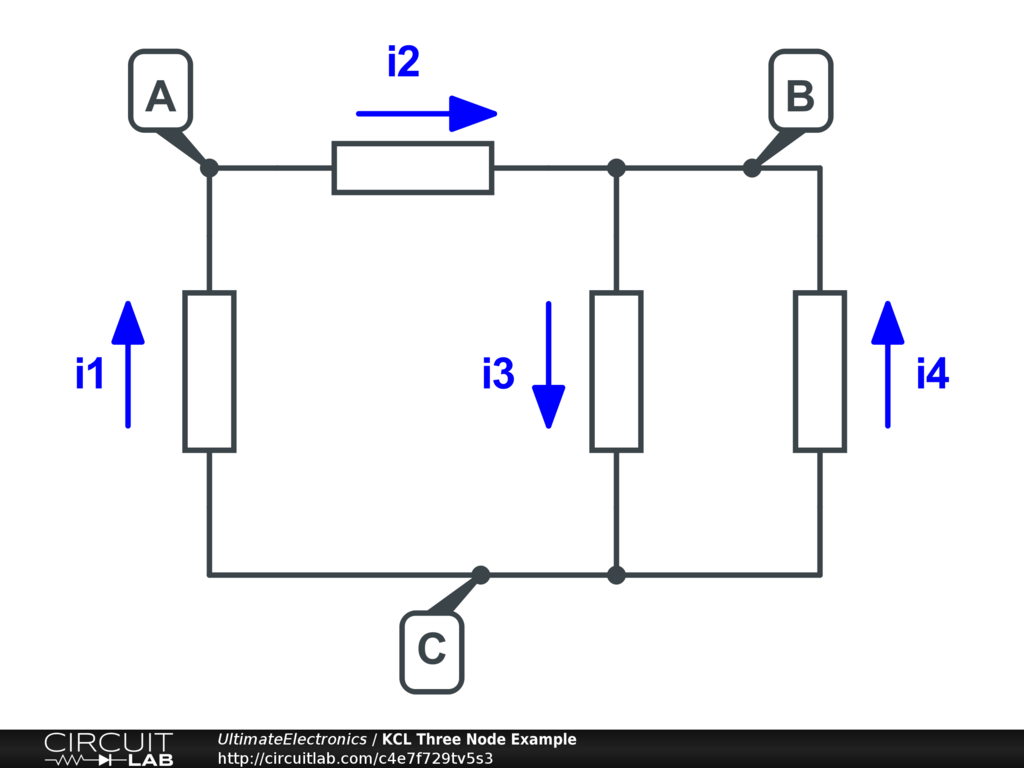We've manually labeled a current arrow through each of the four components shown, and this alone allowed us to write three KCL equations (one at each node A, B, C) describing the structure of the circuit. (Note that while 3 equations were generated, only 2 are linearly independent. Review the KCL section to review these equations.) In fact, in a simple series-connnected set of elements, we might just instantly realize that $i_1 = i_2$ and use only a single variable, as long as the direction was consistent throughout.

The process of manually labeling current variables (and assigning a direction arbitrarily) encompasses much of the reduction processs just described. We can still to ask all possible current questions (i.e. the terminal currents into or out of each component), but now we can refer to these terminal currents in terms of these manually selected current variables, sometimes called branch currents.

A circuit simulator does not generally represent the world in terms of branch currents, but instead computes the terminal currents for various components. However, the two are clearly closely related and can be mapped back and forth by simply identifying the correct branch current that maps to a terminal, and applying a minus sign if the branch current arrow flows "out" of that terminal rather than the conventional definition of terminal currents being positive for flow "into" a terminal.

Relating Branch and Terminal Currents

We can label branch currents in more complicated circuits too, as is often done when solving by hand. For example, we can label our multi-terminal example above: (click to open in a new tab)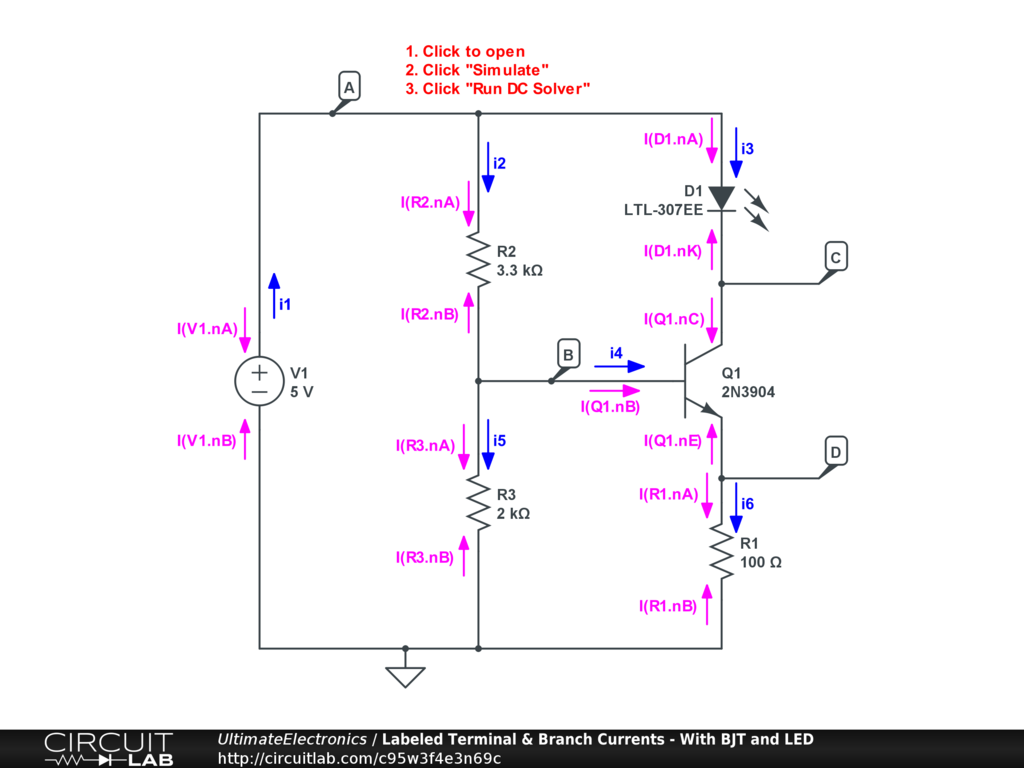We've labeled 6 branch currents, $i_1 \ \text{through} \ i_6$. In general, on a schematic, we wouldn't be so explicit about labeling both the branch currents and the terminal currents, but we're doing it here to be very explicit and show the mapping between the two.

Simply from observing the directions of the arrows along each branch of the circuit we can write many equations mapping branch currents to terminal currents. For example, for $i_1$, we can see that this describes the current flowing through the voltage source V1, and the arrow direction of $i_1$ is opposed to that of $\text{I(V1.nA)}$ but is in the same direction as that of $\text{I(V1.nB)}$. This allows us to write:

\begin{align} i_1 & = - \text{I(V1.nA)} \\ i_1 & = \text{I(V1.nB)} \end{align}

or more simply:

$$i_1 = - \text{I(V1.nA)} = \text{I(V1.nB)}$$

We can repeat this process for each of the 6 branch currents until we've mapped all of the 13 terminal currents to the 6 branch currents:

\begin{align} i_1 & = - \text{I(V1.nA)} = \text{I(V1.nB)} \\ i_2 & = \text{I(R2.nA)} = - \text{I(R2.nB)} \\ i_3 & = \text{I(D1.nA)} = - \text{I(D1.nK)} = \text{I(Q1.nC)} \\ i_4 & = \text{I(Q1.nB)} \\ i_5 & = \text{I(R3.nA)} = - \text{I(R3.nB)} \\ i_6 & = - \text{I(Q1.nE)} = \text{I(R1.nA)} = - \text{I(R1.nB)} \\ \end{align}

Each branch current simply maps to all the terminal currents along that branch, possibly with a minus sign to indicate mismatch of arrow direction. A branch continues as long as no "splits" happen where current can take two paths.

For a circuit with 13 terminals, this lets us answer 26 possible current questions (i.e. current into or out of any terminal) with only 6 underlying variables.

The branch current mapping process tends to take care of nodes where only two terminals meet (such as nodes C and D) automatically because we label them as one contiguous branch, so we assign only a single current variable. For nodes where 3 or more terminals meet (such as A and B), we can apply Kirchhoff's Current Law to the branch currents:

\begin{align} i_1 - i_2 - i_3 & = 0 \\ i_2 - i_4 - i_5 & = 0 \end{align}

Additionally, the branch current mapping process tends to take care of the conservation of charge flow in two-terminal devices as they're labeled with the same branch current. However, for components with three or more terminals, we can generate an extra current summation rule explicitly by observing that the total branches currents in must equal the total branch currents flowing out. For example, for transistor Q1:

$$i_3 + i_4 - i_6 = 0$$

This is an additional, and possibly valuable constraint when solving circuit problems by hand. In circuit simulation software like CircuitLab, however, all multi-terminal devices are effectively modeled internally as a collection of various (possibly nonlinear and interacting) two-terminal devices, so the additional constraint is not necessary.

Labeling and Measurement

Another way to think about the labeling and reduction process is to consider how we would effectively make measurements about a circuit. For this, we'll refer again to the hydraulic analogy of pressurized water flowing through a network of pipes and other components. (For now, we're ignoring the practical issues of precision, etc, and considering only a theoretical model.)

We can ask about the voltage difference (i.e. pressure difference) between any two points in a network, and in a hydraulic system, we could buy differential pressure gauges and install them between every pair of points we care about. However, if we have $N$ points, we have $N^2$ pairs of points (or possibly $\frac {N (N-1)} {2}$ pairs if we ignore $V_{i i} = 0$ and are allowed to measure $V_{a b} = - V_{b a}$). A differential pressure gauge is like a voltmeter with two leads, and it will only show a relative pressure difference. However, that's a lot of differential measurements to make, and these gauges are expensive to buy and install! Instead, we can simply install $N - 1$ differential pressure gauges (taking 1 node as a ground reference), and then subtract when needed to answer any questions about relative differences. For a small hydraulic circuit of only 10 nodes, that's the difference between 45 and 9 gauges to install and read!

Similarly, we could install an ammeter (i.e. a flow rate measurement) at every terminal of every component, but it clearly doesn't make sense to install a flow rate meter on both sides of a single two-terminal component, as the flow rates will be the same assuming no leaks. Instead, we should install just one flow rate measurement per branch. (And, if there are 3+-terminal components we can even be more clever and install fewer than one flow rate per branch by using conservation of flow as shown above.) That's a much smaller number of flow rate meters to purchase, install, and monitor.

Just as we would naturally apply these simplifications in how we measure an electric (or hydraulic) circuit, we apply them in how we set the problem up to solve algebraically because they make that process much simpler.

What's Next

In the next section, Solving Circuit Systems, we'll talk about combining our labeled structural view of a circuit with the individual component-level equations to fully set up and solve circuits of any complexity.